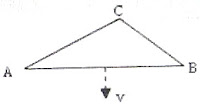## Wednesday, July 25, 2007

### Solution to Practice Questions on Electromagnetic Induction

In the post dated 24th July 2007, two questions on electromagnetic induction were given without solution. The questions with solution are given below as promised in the post:

(1) A search coil in the form of a plane circular copper loop of 10 turns and diameter 2 cm is held within a magnetic field of 20000 gauss, with the plane of the loop perpendicular to the magnetic field. The resistance of the search coil is 0.1 Ω. If the search coil is withdrawn from the magnetic field in a time span of 0.1s, the total charge (in coulomb) and the average current (in ampere) flowing during this time are respectively

(a) 0.1π, 0.5π (b) 0.2π, 2π (c) 0.2π, π

(d) 0.1π, π (e) 0.02π, 0.2π

The charge ‘Q’ induced in the search coil is given by

Q = Flux change/Resistance = nAB/R where ‘B’ is the magnetic flux density and ‘n’, ‘A’ and ‘R’ are respectively the number of turns, area and the resistance of the search coil.

Therefore, Q = [10× π(0.01)2×2]/0.1 = 0.02 π coulomb.

The average current ‘I’ in the coil is given by

I = Q/t = 0.02 π/0.1 = 0.2 π ampere.

(2) A triangular copper loop ABC is moving in its own plane with velocity ‘v’ in a uniform magnetic field acting perpendicular to the plane of the loop. If the direction of motion is perpendicular to the side AB of the loop, an electric field is induced

(a) in AB only

(b) in AC as well as BC, but not in AB

(c) in BC only

(d) in AB, AC and BC

(e) in no side of the loopThere is no change in the magnetic flux linked with the loop and hence the net emf acting in the whole loop is zero. But, since all the conductors AB. AC and BC are moving in a magnetic field, cutting the magnetic field lines, the free charge carriers in them experience magnetic force (Lorentz force) and get shifted towards their ends . So, there are electric fields in all these conductors (generally). In other words, there are induced voltages across AB, AC and BC, but the net voltage in the whole loop is zero since the voltage across AB is equal and opposite to the net voltage across the series combination of AC and BC.

The correct option is (d).

A special case is the possibility of the triangle to be right angled at A or B. If the triangle is right angled at A, the electric field induced across AC is zero. If the triangle is right angled at B, the electric field induced across BC is zero. But the most suitable choice in the above question is still (d).